•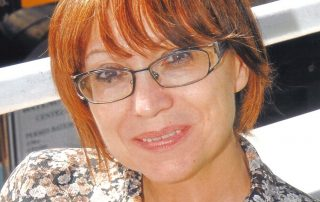## Ilieva, Nevena

Fields of interest/ expertise: • Theoretical and mathematical physics; quantum field theory; field-theoretical methods in condensed-matter theory; • Algorithms for large system modelling and analysis; • Mathematical biology: biocomputing; modelling of biomolecular structure and dynamics; immunoinformatics; protein folding • High-performance computing • History and philosophy of physics

2020-08-11T11:48:59+03:00Monday, 21 October 2019|
•## Zlateva, Plamena

Mathematical modeling and analysis of bioprocesses; mathematical applications in management of nonlinear systems, economics, risk assessment, etc. Mathematical biology, modeling of infectious and chronic diseases, population dynamics.

2020-02-23T18:18:32+02:00Tuesday, 16 July 2019|
•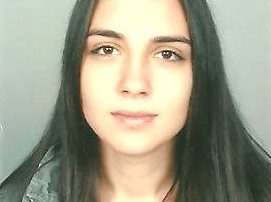## Vucheva, Veselina

2020-06-10T11:31:57+03:00Friday, 27 April 2018|
•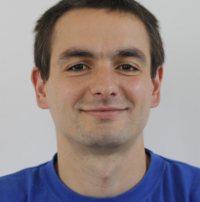## Harizanov, Stanislav

Nonlinear Subdivision; Normal multi-scale transforms for curves and surfaces; Image and Signal Processing; Mathematical Modeling; Constrained Optimization; Primal-Dual Algorithms and their Parallel implementation.

2020-02-23T18:35:22+02:00Friday, 27 April 2018|
•## Stoyanova, Daniela

2018-09-17T21:25:01+03:00Thursday, 26 April 2018|
•## Rashkov, Peter

Mathematical modelling with applications in biology and onclogy, dynamical systems and applications, numerical analysis, harmonic analysis.

2019-07-16T14:37:26+03:00Saturday, 14 April 2018|
•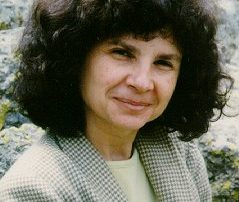## Popova, Evgenija

Numerical and algebraic methods for problems with uncertain (interval) data; Linear algebraic systems involving (non)linear dependencies between interval parameters: methods, software tools, applications; Algebraic extensions (http://www.math.bas.bg/~epopova/directed.html) of the classical interval space; Software tools for problems with uncertain data and/or result verification; Interaction between computer algebra and interval computations; Dynamic and interactive web-accessible mathematical computations and graphics (webComputing) (http://cose.math.bas.bg/webComputing/); Standards for floating-point arithmetic and computer interval arithmetic; Statistical data analysis.

2020-02-20T18:30:43+02:00Friday, 13 April 2018|
•## Markov, Svetoslav

Interval analysis, Numerical methods with verification, Algebraic properties of intervals, errors, convex bodies, stochastic numbers etc., Hausdorff approximations, Mathematical modeling in biology, enzyme kinetics, microbial growth, synaptic transmission, special relativity.

2018-09-17T21:25:49+03:00Tuesday, 10 April 2018|
•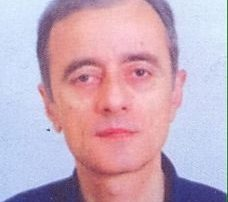## Kyurkchiev, Nikolay

Numerical methods, Approximation theory.

2018-09-17T21:25:44+03:00Tuesday, 10 April 2018|
•## Krastanov, Mikhail

Application of geometrical methods in control theory, Mathematical applications in biosciences, economic and etc.

2018-09-17T21:25:41+03:00Tuesday, 10 April 2018|